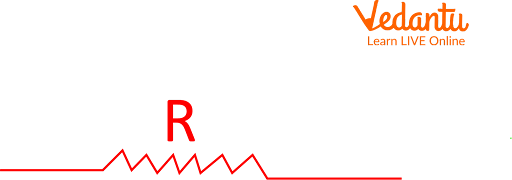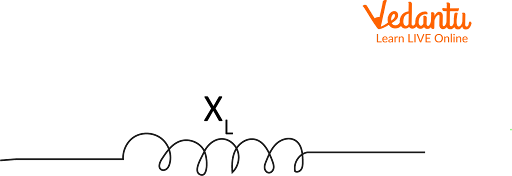Courses
Courses for Kids
Free study material
Free LIVE classes
More

# Difference Between Resistance and Reactance: A Holistic ResearchLIVE
Join Vedantu’s FREE Mastercalss

## An Introduction: Unlocking The Potential of Electricity

Practically speaking, the numerous fundamental electrical components are used while investigating the electrical environment. There are two of them: resistance and reactance. The conductor's characteristics are reactive and resistive. Whenever one starts a journey in Electrodynamics, one of the first concepts encountered is the concept of resistance and reactance along with resistance and reactance examples.

Last updated date: 24th Sep 2023
Total views: 6.3k
Views today: 0.06k

## What is Resistance: An In-Depth Analysis

Resistance is the impediment that a voltage must overcome in order to move a current through a conductor. The applied voltage must be high for the conductor's ends to pass a significant amount of current. That is, according to Ohm's law, the applied voltage (V) must be directly proportional to the current (I) flowing through the conductor, with the resistance (R) of the conductor serving as the constant of proportionality.

$V=I x R$

Conductors have the same resistance whether the current is constant or fluctuating. Using Ohm's law, instantaneous voltage, and current, it is possible to determine resistance for alternating current. The conductor's length (l), cross-sectional area (A), and resistivity () together determine the resistance in Ohms (). Moreover, the conductor's temperature has an impact on resistance as well, with T0 denoting room temperature and also temperature coefficient.

## Reactance in the Conductor:

Reactance is the opposition to a current change. Inductors and capacitors experience reactance when the current is altered. Reactance is thus influenced by the frequency of alternating current passing through an inductor or capacitor. Ohm is its unit.

The capacitor builds up charges when a voltage is applied to its two terminals until the capacitor voltage matches the source voltage. The collected charges are returned to the source at the voltage's negative cycle if the voltage is coming from an alternating current source. The number of charges stored in the capacitor for a brief period of time diminishes as the frequency rises due to the constant charging and discharging time. As a result, the capacitor's resistance to the circuit's current flow reduces as the frequency rises. In other words, the angular frequency () of the alternating current has an inverse relationship with the reactance of the capacitor.

$X_{c} = 1/\omega C$

f is the frequency in Hertz, and C is the capacitance of the capacitor.The impedance of a capacitor, on the other hand, is a negative number. A potential difference is produced when the inductor's current varies. This possible difference is proportional to the rate of fluctuation of the current. As a result, with inductors, the potential difference comes first, followed by the current, by a quarter cycle.

$X_{l} = \omega L=2\pi f L$

XL stands for inductive reactance, L for the inductor's inductance, and f for the alternating current's frequency. The voltage variation will have a different pattern than the current variation due to the resistance of the inductors and capacitors to current variation. This indicates that the alternating current voltage's phase and the alternating current's phase are different. While capacitive reactance causes the current phase to lead, inductive reactance has the tendency which causes the current phase to lag behind the voltage phase. In perfect components, the amount of this can be upto 90 degrees.

## Symbolic Representation of Resistance and Reactance:

### For Resistance:Resistor

### For Inductive Reactance:Inducting coil

### For Capacitive Reactance:Capacitor

## Difference Between Resistance and Reactance:

### Reactance

Definition

Resistance is a property of a substance that prevents or limits the flow of current in a circuit.

Reactance is a property of a material that opposes a change in current in the circuit.

Formula

$V=I x R$ where R is the resistance

$X_{c} = 1/\omega C$ and X_{l} = \omega L=2\pi f L$Where Xc and XL are Capacitive reactance and inductive reactance Factors influencing the magnitude Resistance is affected by four factors namely length of the conductor(l), Area of the cross section(A), Temperature(T) and resistivity($\rho$) The frequency of the alternating current affects reactance along with capacitance in the case of capacitive inductance and inductance in the case of inductive reactance. Phase difference between voltage and current Phase difference is zero in the solely resistive circuit. In capacitive reactance, potential difference leads current by 900 in phase and in inductive reactance, potential difference lags current by 900 in phase. Area of significance In a resistive circuit, electric power is lost as heat. This electrical energy is kept in reserve in a reactive circuit via inductive and capacitive components. 6. Power consumption Real power is consumed in resistive circuit Power is not fully utilized in this circuit. ## Summary It is crucial to differentiate between resistance and reactance because these are two key ideas in the chapter on current electricity. For current to flow through electrical components like resistors, capacitors, and inductors, an impedance—a complex number—must be created. Pure resistors have an impedance with a real value called resistance, but ideal inductors and capacitors have an impedance with an imaginary value called reactance. Reactance only occurs on variable currents, whereas resistance exists on both direct and alternating currents, causing the component to resist the change in current. Reactance changes with AC frequency, whereas resistance is frequency-independent. Phase shifts between the current and voltage phases are also brought on by reactance. This article explains resistance and reactance examples along with a detailed symbolic representation of resistance and reactance. ## FAQs on Difference Between Resistance and Reactance: A Holistic Research 1. What is resistance and reactance? Resistance is the challenge that the voltage encounters when directing a current through a conductor. The applied voltage to the conductor's ends must be high in order for it to pass a big current. That is, in accordance with Ohm's rule, the applied voltage (V) must be inversely proportional to the current (I) flowing through the conductor, with resistance (R) serving as the proportionality constant. On the other hand, reactance is opposition to a current change. Inductors and capacitors experience reactance when the current is altered. As a result, the frequency of the alternating current passing through an inductor or capacitor has an impact on reactance. Ohm is its unit. 2. Using formula, explain capacitive and inductive reactance? Capacitive reactance is a measurement of the capacitor's resistance to alternating current. Capacitive reactance is measured in Ohms.$X_{c} = 1/\omega C$Inductive reactance, which resembles the resistance to direct current (DC) in a resistance, is the characteristic of an inductive coil that opposes the change in alternating current (AC) through it.$X_{l} = \omega L=2\pi f L\$

3. What is the phase difference between potential difference and current in a circuit comprising only (i) resistor, (ii) capacitor and (iii) inductor coil?

For a resistor, resistance produced has no effect on phase difference between potential difference and current i.e., phase difference is zero. For capacitors which produce  capacitive reactance, potential difference leads current by 900  in phase and  for an inductor coil which produces inductive reactance, potential difference lags current by 900 in phase.